MON - FRI 8AM - 5PM

# What’s the Return on Investment (ROI) of an Automatic Lubrication System?

An automatic lubrication system provides many benefits, but how do you calculate its ROI?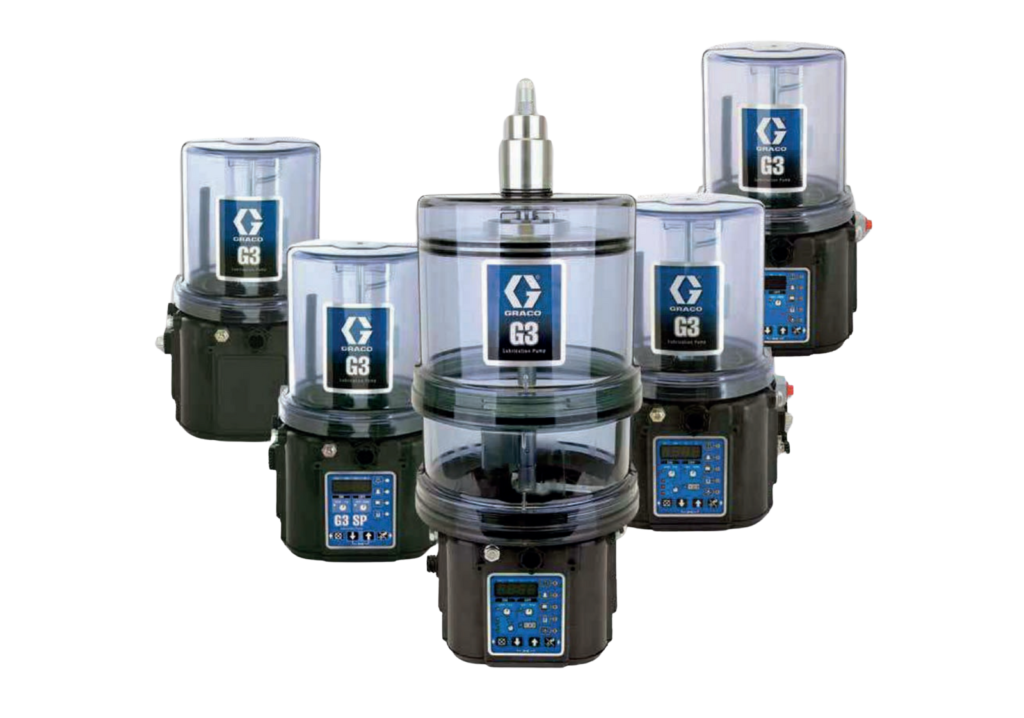## How to Optimize Running Time?

Machine downtime has an immediate impact on the return on investment (ROI) and the profit of construction and mining equipment. An investment in an automatic lubrication system for a typical machine such as the Caterpillar 320 excavator has an ROI of as little as nine months.

Construction equipment such as excavators, wheel loaders, articulated dump trucks, and similar machines are capital-intensive products and need to work as much as possible in order to pay back the investment. The return on investment (ROI) is a performance measure that expresses the efficiency and the payback period of an investment. The efficiency of construction equipment is directly related to its running time. To increase the ROI you need to optimize the running time of the machine.

The best way to optimize machine running time is to minimize the service and maintenance hours. Being a frequently performed and time-consuming task, lubrication is the most decisive factor. An automatic lubrication system, which automatically lubricates equipment while it is running, is the best way to optimize the running time of off-road machines.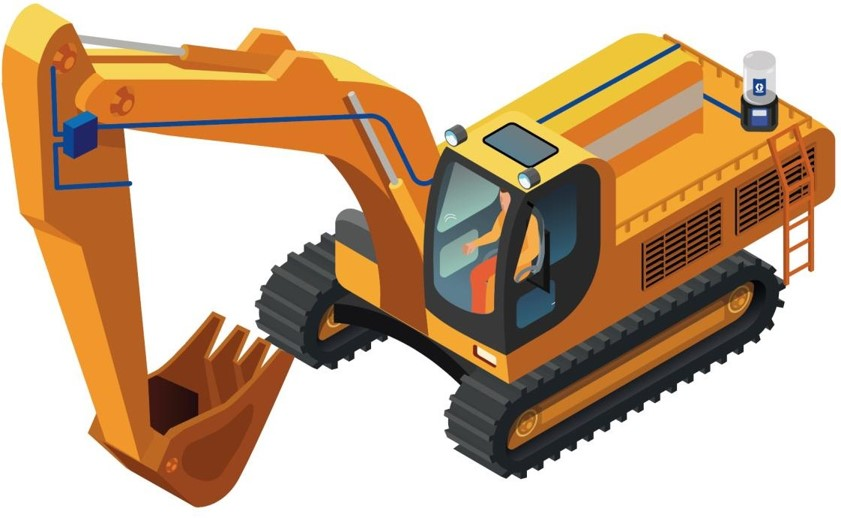## How to calculate ROI of lubrication systems?

In order to calculate the ROI for an automatic lubrication system, you need to compare the cost of the automatic lubrication system (ALS) and the cost involved in manual lubrication.

Return on investment = cost of ALS installed on the machine / cost of manual lubrication per year

The cost for an automatic lubrication system for a typical machine such as the Caterpillar 320 excavator varies depending on options and features but is between \$3,000 and \$4,500.

The cost of manual lubrication can be split into:

Costs of downtime – that is profits lost due to unavailability of the machine
Labor costs – the wages of technicians who perform manual lubrication

Manual lubrication costs must also factor in the duration and frequency of greasing activities as a ratio of the total operation days per year when the machine is used.

## 5 Steps to Calculating the Annual Cost of Manual Lubrication

To calculate the manual lubrication costs, we will split the identified parameters related to manual lubrication using the example of a Caterpillar 320 excavator. This machine is used throughout the year at a construction site and is lubricated daily by an onsite technician.

Step 1: How to calculate costs of downtime

To calculate the cost of machine downtime during manual lubrication, we will estimate the value of profits lost using the rental price of a machine. That is the income that would have been accrued if the machine had been in operation.

Cost of machine downtime = (rental) cost of machine per hour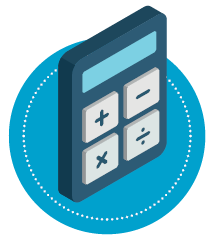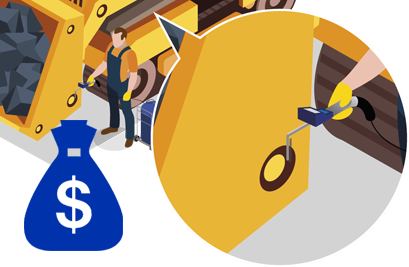Step 2: How to calculate labor costs

Next, we calculate the labor cost per hours of the employee who does the lubrication.
Cost of labor for manual lubrication = Labor cost per hour

To make this part of the calculation at a later stage easier, we can combine the first two hourly costs into one: cost of machine downtime + cost of labor for manual lubrication = (cost of machine + labor cost) per hour

For this scenario, we will estimate that the rental cost per hour for a 22-ton excavator is \$56 and the labor cost per hour is \$25.
If we use these figures in the equation, we get: \$56 + \$25 per hour.

Step 2: How to calculate labor costs

Next, we calculate the labor cost per hours of the employee who does the lubrication.
Cost of labor for manual lubrication = Labor cost per hour

To make this part of the calculation at a later stage easier, we can combine the first two hourly costs into one: cost of machine downtime + cost of labor for manual lubrication = (cost of machine + labor cost) per hour

For this scenario, we will estimate that the rental cost per hour for a 22-ton excavator is \$56 and the labor cost per hour is \$25.
If we use these figures in the equation, we get: \$56 + \$25 per hour.Step 3: How to calculate the duration of manual lubrication?

The time needed to do the manual lubrication is based on the number of lubrication points and the time needed to lubricate each point.

Duration of manual lubrication = Lubrication points * Time to lubricate one point

The Caterpillar 320 excavator has 20 lubrication points. Assuming it takes 1 minute to lubricate one point (i.e. allocate the grease fitting, clean it, connect the manual grease gun, dispense grease, disconnect the gun, and clean grease fitting again), the total time to lubricate the machine will be 20 minutes.  Using the conversion that 1min = 1/60, means the duration of manual lubrication = 20 points * (1/60).

However, a study done by a major oil company estimates that 3 minutes is the average time needed to manually lubricate one grease point.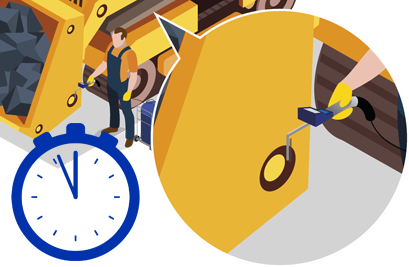Step 4: How to calculate the frequency

The frequency describes how often per day, week, etc, manual lubrication is done. It usually varies depending on the manufacturer’s recommended service intervals and environmental conditions. In reality, the frequency of manual lubrication depends on technicians’ maintenance activities.
Frequency = 1 “once” / Days between manual lubrication

Step 5: How to calculate the yearly machine usage in days

For total operation days per year, we assume that the machine is used for 220 standard working days (minus weekends and holidays).

In our example with the Caterpillar 320, we consider that the machine is lubricated once each day throughout the year (220 operation days). Thus, going back to our equations, we can calculate as follows: Frequency = 1/1 and Days per year = 220.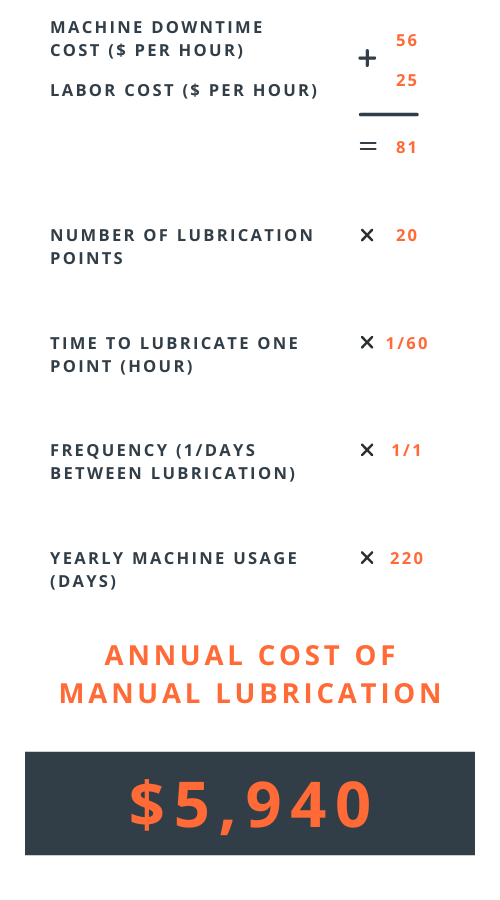#### Yearly cost of manual lubrication

Having determined the annual cost for the downtime of a 22-ton excavator like the Caterpillar 320 with an hourly rental fee of \$56, \$25 labor cost, 20 lubrication points, 1 minute to lubricate each point, daily lubrication and a yearly machine usage of 220 days, we can use this to calculate the return on investment.

Cost of manual lubrication per year = (cost of machine + labor cost) * Duration of manual lubrication * Frequency * Days per year

Cost of manual lubrication = (\$56 per hour + \$25 per hour) * 20 points * (1/60) * (1/1) * 220 = \$5,940

## Conclusion

Going back to our first equation gives us the following: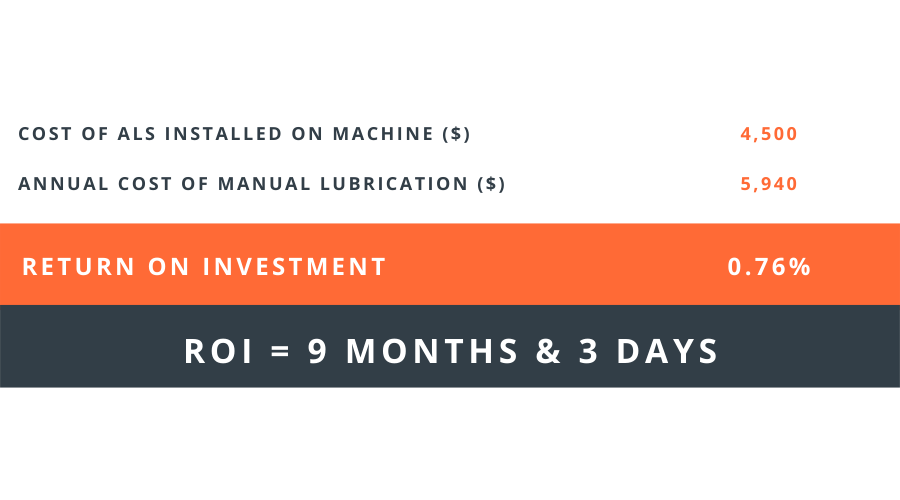This gives us a return on investment of 9 months and 3 days, taking the cost of an automatic lubrication system to be \$4,500. It means the investment of an automatic lubrication system is earned back in its entirety after 9 months and 3 days. After this period, your investment will generate nothing but profit.### SOCIAL MEDIA